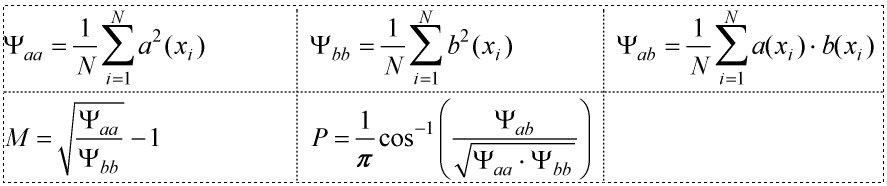# Sprague-Geers Metric for Data Similarity

This section defines and provides examples of the Sprague-Geers Metric for Data Similarity.

## Definition

Consider two signals a(xk) and b(xk), k=1…N. You are interested in computing a metric that shows the similarity of these signals. Use the following steps to compute the Sprague-Geers metric:
1. Calculate the metric M for magnitude difference and P for phase difference as follows:2. Calculate the combined metric C as follows:

$C=1-\sqrt{{M}^{2}+{P}^{2}}$

C=1 is a perfect match. Metric C is what we want to show.
Note: $\sqrt{{M}^{2}+{P}^{2}}$ is known as the Sprague-Geers Combined Metric.

## Example 1: Signals with the same overall magnitude, but drastically different slopes

Ψaa=143.50, Ψbb=143.50, Ψab=77.00, M+0.00, P=0.319714, C=0.680286

## Example 2: Two very similar signals displaced in the y direction by 5%

Ψaa=0.50, Ψbb=0.51, Ψab=0.50, M=0.009852, P=0.044719, C=0.954208

## Example 3: A synthesized MIT example for Dynamic Stiffness

Ψaa=3.39E+5, Ψbb=3.77E+5, Ψab=3.57E+5, M=0.051164, P=0.026831, C=0.942227

## Example 4: A synthesized MIT example for Loss Angle

Ψaa=1.39628E+1, Ψbb=6.67958, Ψab=9.59216, M=0.445811, P=0.037025, C=0.552654